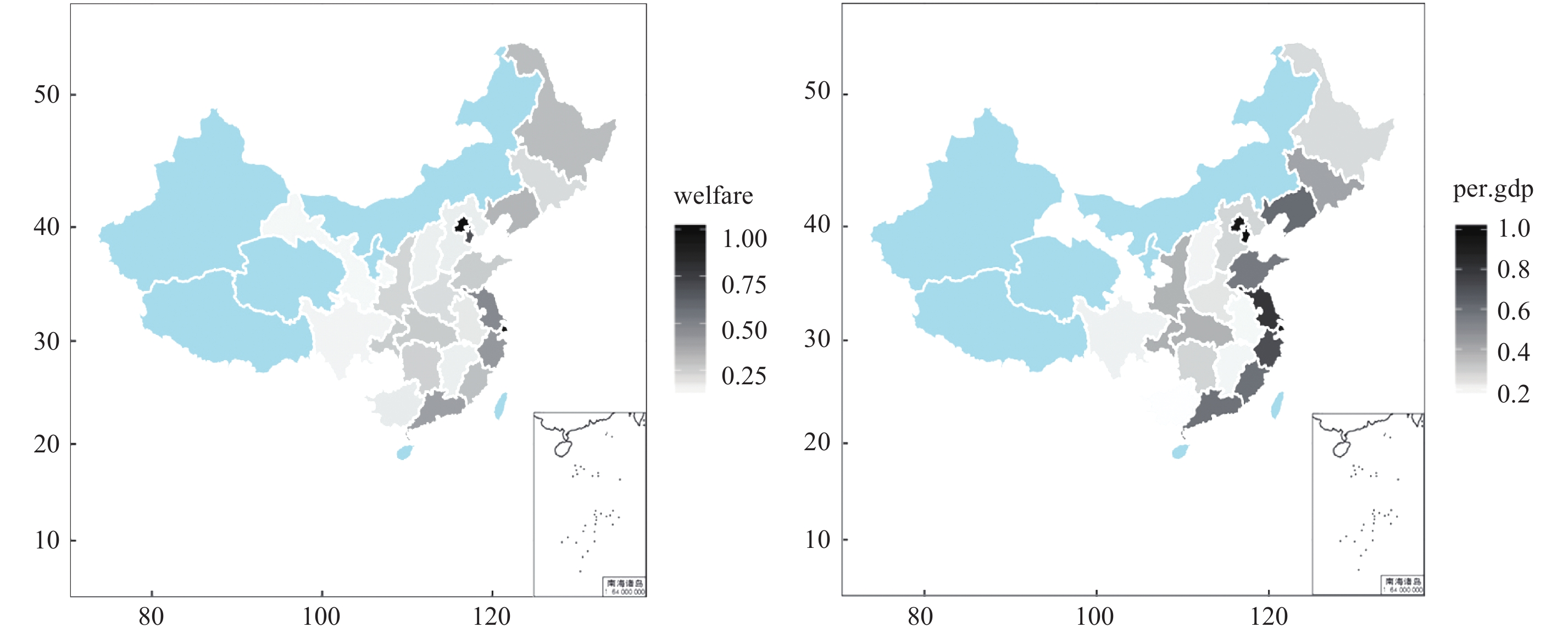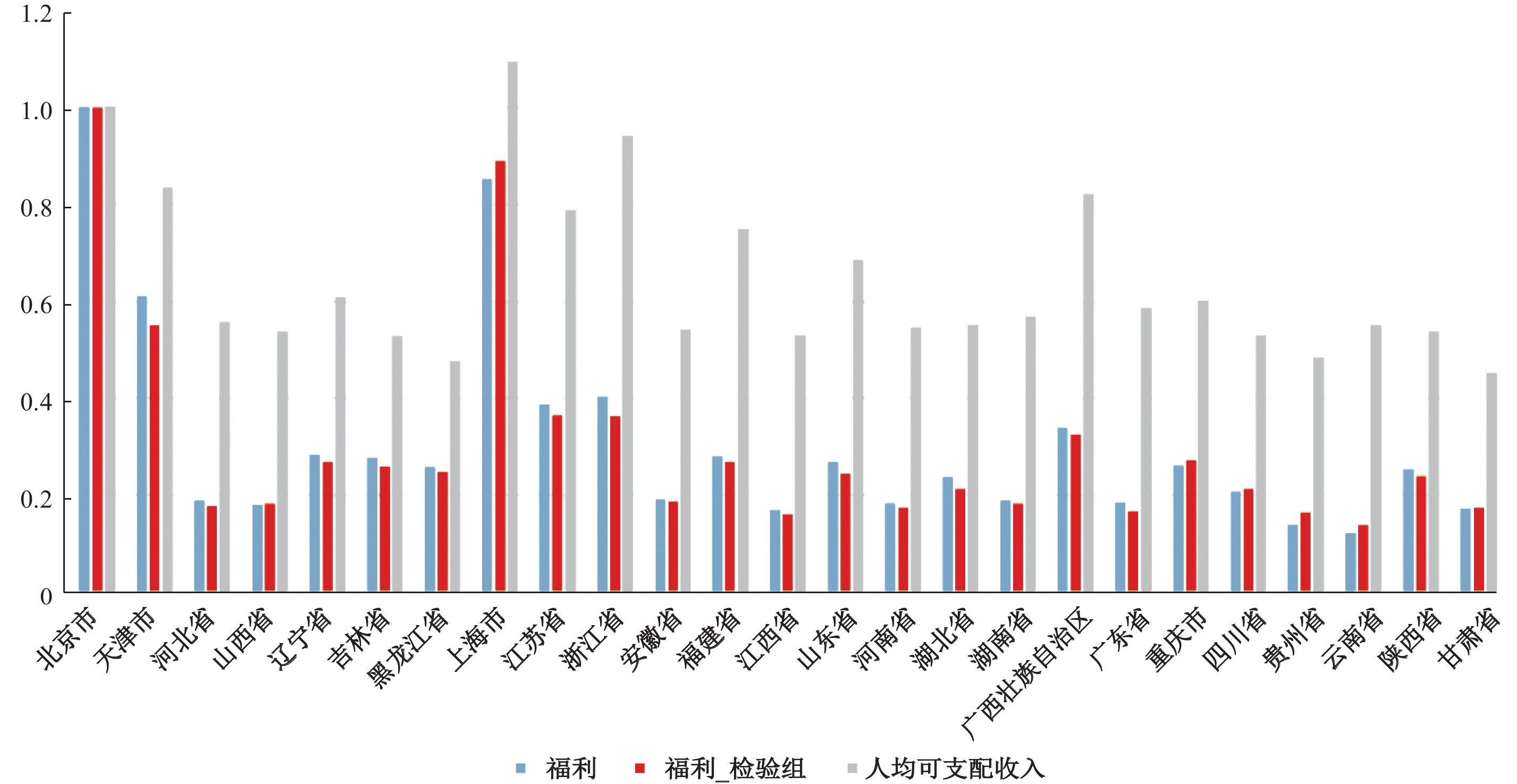﻿ 中国分区域城镇居民福利水平测度
 财经研究2018, Vol. 44Issue (10): 111-1240

#### 文章信息财经研究2018年44卷第10期

Chen Zhihong, Li Yang.

A measurement of chinese urban residents’ welfare by region

Journal of Finance and Economics, 2018, 44(10): 111-124.

### 文章历史《财经研究》
2018第44卷第10期

A Measurement of Chinese Urban Residents’ Welfare by Region
Chen Zhihong, Li YangSchool of International Trade and Economics，University of International Business and Economics，Beijing 100029，China
Summary: With China’s continuous deepening of urbanization, local governments of different provinces and cities have adopted competitive policies to attract more talented workers. In first-tier cities, one can have a higher payment, a better educational environment and more medical resources at the cost of intensive work, expensive housing, pollution and traffic. This tradeoff lends importance to the task of measuring welfare to live in different cities. While traditionally welfare is highly correlated with GDP per capita, deviations are often large.　　This paper chooses a more comprehensive statistic to measure the well-being of people in a province by incorporating consumption, leisure, mortality, and inequality in this statistic. Utilizing the detailed household-level information from the 2010-2014 China Family Panel Studies （CFPS） data, we calculate the whole life welfare of Chinese urban residents in different provinces. Our empirical results show that: Firstly, measurement using GDP per capita only might overestimate or underestimate the welfare inequality although GDP is highly correlated with the welfare. Secondly, first-tier cities like Beijing and Shanghai are strikingly far ahead of other provinces in terms of comprehensive welfare, even relatively more developed provinces such as Zhejiang and Jiangsu achieve the level of as small as 1/3 of Beijing, and this gap in welfare between first-tier cities and other provinces has been increasingly widened. Thirdly, public finance transfer can reduce the inequality in welfare. Therefore, the comprehensive welfare measurement demonstrates less inequality than the GDP per capital in other regions in China. Fourthly, welfare grows slower than GDP per capita, but shrinks much faster when the economy goes down. 　　This paper contributes to the literature by taking a more theory-based measurement and more micro-foundation data to provide comparisons of welfares among different provinces. The results have practical implication for people who choose where to work and live to achieve better well-being.
Key words: welfare    lifelong utility    regional comparison    structural model    micro data

“北上广深”是中国广大年轻人追逐梦想的地方，但随着一线城市人口的逐渐饱和，定居成本的不断攀升，人们逃离“北上广”的呼声越来越强烈。是选择在“北上广”打拼，还是到二三线城市过较为轻松的生活？这是广大“北漂”和“海漂”内心都在挣扎的问题。一方面，一线城市的就业机会多、工资待遇高、教育和医疗条件更好，生活设施也便利；另一方面，大城市的交通拥堵、工作压力大、近年来房价飙升，加上最近几年北方雾霾天气严重，户口和购车摇号的门槛越来越高不可攀，定居在一线城市的压力从来没有像今天这么大，承受不住生活压力的年轻人很多选择了离开。如果选择逃离“北上广深”，到家乡的二三线城市定居，相比留在“北上广”，一生会损失多少福利？这是本文尝试回答的问题。

 $U = E\mathop \sum \limits_{a = 1}^{100} {\beta ^a}u\left( {{C_a},{l_a}} \right)S\left( a \right)$ (1)

 ${U_i}\left( \lambda \right) = {E_i}\mathop \sum \limits_{a = 1}^{100} {\beta ^a}u\left( {\lambda {C_{ai}},{l_{ai}}} \right){S_i}\left( a \right)$ (2)

 ${U_{bj}}\left( {{\lambda _i}} \right) = {U_i}\left( 1 \right)$ (3)

 $u\left( {C,l} \right) = \bar u + logc + v\left( l \right)$ (4)

 $v\left( l \right) = - \frac{{\theta \epsilon }}{{1 + \epsilon }}{\left( {1 - l} \right)^{\frac{{1 + \epsilon }}{\epsilon }}}$ (5)

 $\bar \omega _{ja}^j = \frac{{\omega _{ja}^j}}{{\mathop \sum \nolimits_{j = 1}^{N_a^i} \omega _{ja}^i}}$ (6)

 ${U_i} = \mathop \sum \limits_{a = 1}^{100} {\beta ^a}S_a^i\mathop \sum \limits_{j = 1}^{N_a^i} \bar \omega _{ja}^ju\left( {c_{ja}^i{e^{ga}},l_{ja}^i} \right)$ (7)

 ${U_{bj}}\left( {{\lambda _i}} \right) = \mathop \sum \limits_{a = 1}^{100} {\beta ^a}S_a^{bj}\left[ {u_a^{bj} + log\left( {{\lambda _i}} \right)} \right]$ (8)

 $u_a^{bj} = \bar u + ga + \mathop \sum \limits_{j = 1}^{N_a^{bj}} \bar \omega _{ja}^{bj}\left[ {{\rm log}\left( {c_{ja}^{bj}} \right) + v\left( {l_{ja}^{bj}} \right)} \right]$ (9)

 ${\rm log}\left( {{\lambda _i}} \right) = \frac{1}{{\mathop \sum \nolimits_a {\beta ^a}S_a^{bj}}}\mathop \sum \limits_a {\beta ^a}\left[ {\left( {S_a^i - S_a^{bj}} \right)u_a^i + S_a^{bj}\left( {u_a^i - u_a^{bj}} \right)} \right]$ (10)

 $s_a^{bj} = \frac{{{\beta ^a}S_a^{bj}}}{{\mathop \sum \nolimits^ {\beta ^a}S_a^{bj}}}$
 $\Delta s_a^i = \frac{{{\beta ^a}\left( {S_a^i - S_a^{bj}} \right)}}{{\mathop \sum \nolimits^ {\beta ^a}S_a^{bj}}}$

 ${\rm log}\left( {{\lambda _i}} \right) = \sum\nolimits_a {\Delta s_a^iu_a^i} + \sum\nolimits_a {s_a^{bj}} \left( {u_a^i - u_a^{bj}} \right)$ (11)

 ${\rm log}\left( {{\lambda _i}} \right) = \mathop \sum \limits_a \Delta s_a^iu_a^i + \mathop \sum \limits_a s_a^{bj}\left( {\mathop \sum \limits_{j = q}^{N_a^i} \bar \omega _{ja}^i\left[ {{\rm log}\left( {c_{ja}^i} \right) + v\left( {l_{ja}^i} \right)} \right] - \mathop \sum \limits_{j = q}^{N_a^{bj}} \bar \omega _{ja}^{bj}\left[ {{\rm log}\left( {c_{ja}^{bj}} \right) + v\left( {l_{ja}^{bj}} \right)} \right]} \right)$

 ${{\bar c}_i} = \mathop \sum \limits_a s_a^{bj}\mathop \sum \limits_{j = 1}^{N_a^i} \bar \omega _{ja}^ic_{ja}^i{e^{ga}}$
 ${{\bar l}_i} = \mathop \sum \limits_a s_a^{bj}\mathop \sum \limits_{j = 1}^{N_a^i} \bar \omega _{ja}^il_{ja}^i$
 $E{\rm log}{c_i} = \mathop \sum \limits_a s_a^{bj}\mathop \sum \limits_{j = 1}^{N_a^i} \bar \omega _{ja}^i{\rm log}\left( {c_{ja}^i{e^{ga}}} \right)$
 $Ev\left( {{l_i}} \right) = \mathop \sum \limits_a s_a^{bj}\mathop \sum \limits_{j = 1}^{N_a^i} \bar \omega _{ja}^iv\left( {l_{ja}^i} \right)$
 ${\rm log}\left( {{\lambda _i}} \right) = \sum\nolimits_a {\Delta s_a^iu_a^i} + E{\rm log}{c_i} - E{\rm log}{c_{bj}} + Ev\left( {{l_i}} \right) - Ev\left( {{l_{bj}}} \right)$ (12)

 \begin{aligned}{{\rm log}\frac{{{\lambda _i}}}{{\widetilde {{y_i}}}} = }&{\mathop \sum \limits_a \Delta s_a^iu_a^i} \quad \quad \quad \quad\quad \; \quad \quad \quad \quad \quad \quad \text{生命}\\{}&{ + \log \frac{{{{\bar c}_i}}}{{{y_i}}} - \log \frac{{{{\bar c}_{bj}}}}{{{y_{bj}}}}} \quad \quad \quad \quad\quad \; \quad \quad\quad \text{消费}\\{}&{ + v\left( {{{\bar l}_i}} \right) - v\left( {{{\bar l}_{bj}}} \right)} \quad \quad \quad \quad \quad \quad \quad \ \, \quad\quad\text{闲暇}\\{}&{ + E{\rm log}{c_i} - {\rm log}{{\bar c}_i} - \left( {E{\rm log}{c_{bj}} - {\rm log}{{\bar c}_{bj}}} \right)} \quad \text{消费不平等}\\{}&{ + Ev\left( {{l_i}} \right) - v\left( {{{\bar l}_i}} \right) - \left( {Ev\left( {{l_{bj}}} \right) - v\left( {{{\bar l}_{bj}}} \right)} \right)} \quad \quad \text{闲暇不平等}\end{aligned} (13)

 省份 福利 收入 福利/收入对数 福利分解 寿命 消费/收入 闲暇 消费不平等 闲暇不平等 北京市 1 1 0 0 0 0 0 0 83.481 1.166 0.867 1.498 0.211 天津市 0.699 1.052 −0.409 −0.049 −0.448 0.014 0.061 0.013 83.051 0.745 0.874 1.458 0.208 河北省 0.178 0.4 −0.809 −0.408 −0.306 −0.02 −0.025 −0.051 80.316 0.858 0.857 1.402 0.228 山西省 0.13 0.351 −0.989 −0.391 −0.301 −0.029 −0.188 −0.08 80.406 0.863 0.852 1.407 0.238 辽宁省 0.32 0.652 −0.713 −0.325 −0.305 −0.019 −0.024 −0.039 81.11 0.86 0.857 1.454 0.225 吉林省 0.219 0.502 −0.831 −0.204 −0.42 −0.04 −0.07 −0.098 81.903 0.766 0.847 1.368 0.242 黑龙江省 0.278 0.392 −0.346 −0.325 0.102 0.023 −0.132 −0.014 81.12 1.291 0.879 1.384 0.215 上海市 0.91 0.974 −0.068 −0.055 0.006 −0.007 −0.027 0.015 83.26 1.173 0.863 1.491 0.205 江苏省 0.457 0.819 −0.584 −0.218 −0.209 −0.063 −0.029 −0.065 81.865 0.946 0.837 1.483 0.233 浙江省 0.44 0.73 −0.507 −0.155 −0.215 −0.047 −0.018 −0.073 82.454 0.94 0.844 1.445 0.234 安徽省 0.163 0.344 −0.75 −0.23 −0.243 −0.085 −0.062 −0.129 81.686 0.914 0.827 1.398 0.251 福建省 0.261 0.635 −0.888 −0.195 −0.504 −0.062 −0.081 −0.047 81.976 0.705 0.837 1.433 0.227 江西省 0.161 0.347 −0.768 −0.425 −0.275 −0.07 0.039 −0.038 80.278 0.886 0.834 1.336 0.223 山东省 0.273 0.609 −0.802 −0.283 −0.394 −0.042 −0.023 −0.059 81.335 0.786 0.846 1.415 0.23 河南省 0.193 0.371 −0.651 −0.324 −0.224 −0.015 −0.062 −0.026 80.959 0.932 0.859 1.398 0.22 湖北省 0.287 0.471 −0.496 −0.169 −0.243 −0.019 −0.017 −0.048 82.258 0.914 0.857 1.41 0.227 湖南省 0.232 0.403 −0.553 −0.256 −0.268 0.011 −0.045 0.005 81.497 0.892 0.872 1.464 0.212 广东省 0.377 0.635 −0.521 −0.248 −0.151 −0.023 −0.054 −0.045 81.703 1.002 0.855 1.462 0.225 广西 0.203 0.331 −0.487 −0.208 −0.086 −0.118 −0.047 −0.027 81.879 1.069 0.814 1.437 0.222 重庆市 0.355 0.479 −0.298 −0.118 −0.16 0.002 −0.017 −0.005 82.628 0.994 0.868 1.459 0.215 四川省 0.21 0.351 −0.513 −0.139 −0.12 −0.016 −0.183 −0.055 82.441 1.034 0.859 1.455 0.231 贵州省 0.14 0.264 −0.634 −0.329 −0.05 −0.07 −0.107 −0.079 81.147 1.109 0.834 1.419 0.236 云南省 0.109 0.273 −0.915 −0.75 0.021 −0.128 0.003 −0.06 78.032 1.19 0.811 1.393 0.232 陕西省 0.28 0.469 −0.516 −0.196 −0.225 −0.009 −0.052 −0.034 81.907 0.931 0.862 1.474 0.225 甘肃省 0.185 0.264 −0.359 −0.264 −0.002 −0.019 −0.015 −0.058 81.547 1.163 0.857 1.402 0.231

（一）城镇居民福利水平分解及分区域比较图 1 2014年中国各省份人均政府消费和人均GDP图 2 2014年中国分区域城镇居民的福利和人均GDP地图

（二）城镇居民福利增长率

GDP增长率是衡量地区经济发展的重要指标，而经济的发展是否转化为居民真实的福利则更为重要。很多人抱怨收入跑不过CPI，福利增速比不上经济增速，本文对2010年、2012年和2014年共三年的数据进行了福利水平测算，得出这三年福利水平增长的柱状图见图3图 3 中国2010年、2012年和2014年分区域的城镇居民福利指数图 4 中国2010−2014年分区域城镇居民福利指数增长率和GDP增长率对比图 5 2010年城镇居民福利稳健性检验和人均可支配收入对比

① 因篇幅限制，2010年和2012年福利水平分解结果未予列出，如有需要可向作者索取。

  方福前, 吕文慧. 中国城镇居民福利水平影响因素分析——基于阿马蒂亚•森的能力方法和结构方程模型[J]. 管理世界, 2009(4): 17–26.  李连友, 宋泽, 刘子兰. 城镇移民生活福利水平研究[J]. 中国人口科学, 2014(6): 62–70.  杨爱婷, 宋德勇. 中国社会福利水平的测度及对低福利增长的分析——基于功能与能力的视角[J]. 数量经济技术经济研究, 2012(11): 3–17.  叶静怡, 王琼. 进城务工人员福利水平的一个评价——基于Sen的可行能力理论[J]. 经济学（季刊）, 2014(4): 1323–1344.  余谦, 高萍. 中国农村社会福利指数的构造及实测分析[J]. 中国农村经济, 2011(7): 63–71.  袁方, 史清华. 不平等之再检验: 可行能力和收入不平等与农民工福利[J]. 管理世界, 2013(10): 49–61.  Aguiar M, Mark B. Has consumption inequality mirrored income inequality?[J]. American Economic Review, 2015, 105(9): 2725–2756. DOI:10.1257/aer.20120599  Becker G S, Philipson T J, Soares R R. The quantity and quality of life and the evolution of world inequality[J]. The American Economic Review, 2005, 95(1): 277–291. DOI:10.1257/0002828053828563  Córdoba J C, Verdier G. Inequality and growth: Some welfare calculations[J]. Journal of Economic Dynamics and Control, 2008, 32(6): 1812–1829. DOI:10.1016/j.jedc.2007.06.015  Fleurbaey M, Gaulier G. International comparisons of living standards by equivalent incomes[J]. The Scandinavian Journal of Economics, 2009, 111(3): 597–624. DOI:10.1111/sjoe.2009.111.issue-3  Jones C I, Klenow P J. Beyond GDP? Welfare across countries and time[J]. The American Economic Review, 2016, 106(9): 2426–2457. DOI:10.1257/aer.20110236  Nordhaus W D, Tobin J. Is growth obsolete?[A]. Nordhaus W D, Tobin J. Economic research: Retrospect and prospect: Economic growth[M]. New York: National Bureau of Economic Research, 1972: 1—80.  Ravallion M. Mashup indices of development[J]. The World Bank Research Observer, 2011, 27(1): 1–32.  Sen A. Equality of what?[A]. McMurrin S M. Tanner Lectures on Human Values[M]. Cambridge: Cambridge University Press, 1980.  Sen A. Inequality Reexamined[M]. Oxford: Oxford University Press, 1992.